# Bar induction

An inductive way of reasoning, used in intuitionistic mathematics (cf. Intuitionism), which may be described as follows. Let certain properties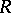andbe defined on finite tuples (cf. Tuple) of natural integers, such that 1) the propertyis decidable, i.e. it is possible to effectively find out whether it is fulfilled on any tuple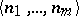or not; 2) for any choice sequenceit is possible to find a tuple of the form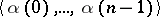for whichis fulfilled. Moreover, if 2) is fulfilled, one says that"bars" the empty tuple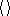(hence the name); 3) for any tupleof natural integers, if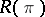, then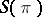(the so-called basis of the bar induction); and 4) ifis a tuple such that for any natural numberone has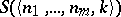, then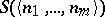; this property is known as the step of the bar induction.

If the conditions 1) through 4) above are met, one can deduce from the principle of bar induction that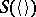.

The use of bar induction was proposed by L.E.J. Brouwer as an intuitionistic method of reasoning which indicates that the collection of free choice sequences is incomplete and to some extent uncountable. It was demonstrated, in particular, by S.C. Kleene and, independently, by A.A. Markov that it follows from the principle of bar induction (in fact, even from a corollary of bar induction, the fan theorem) that not all choice sequences are recursive (cf. Fan).

The forms of bar induction which one started to utilize in the foundations of mathematics in the 1960's do not deal with tuples of natural numbers, but rather with tuples of more complex objects, such as tuples of choice sequences.

Bar induction can be written down in the language of formal intuitionistic mathematical analysis as follows: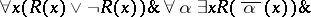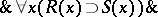How to Cite This Entry:
Bar induction. A.G. Dragalin (originator), Encyclopedia of Mathematics. URL: http://encyclopediaofmath.org/index.php?title=Bar_induction&oldid=12849
This text originally appeared in Encyclopedia of Mathematics - ISBN 1402006098